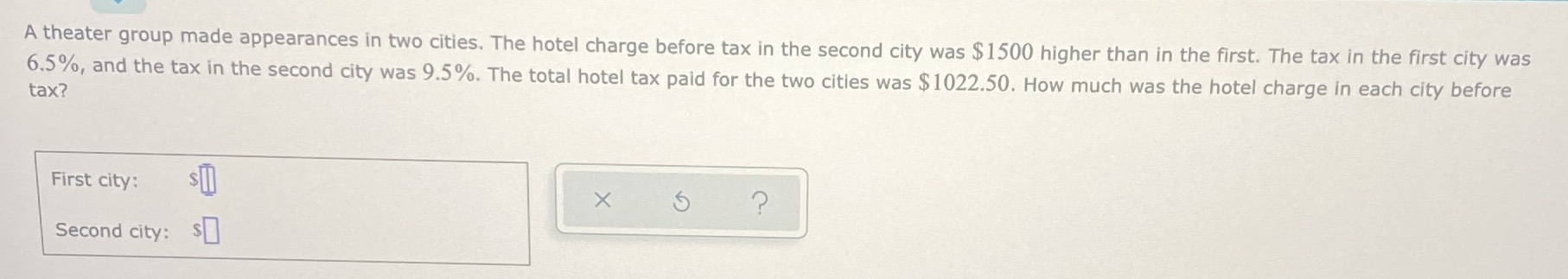### Still have math questions?

Algebra
QuestionA theater group made appearances in two cities. The hotel charge before tax in the second city was $$\ 1500$$ higher than in the first. The tax in the first city was $$6.5 \%$$ , and the tax in the second city was $$9.5 \%$$ . The total hotel tax paid for the two cities was $$\ 1022.50$$ . How much was the hotel charge in each city before tax?

First city: $$\ \square$$

Second city: $$\ \square$$

let the hotel charge in first city before tax be x

hotel charge in second city before tax = x+1500

tax paid in first city = 6.5%of x

tax paid in second city = 9.5% of  (x+15)

total tax = 1022.50

$$\frac{6.5}{100}x+ \frac{9.5}{100}(x+ 1500)$$ = 1022.5

x= 6381.72

so

hotel charge in first city before tax =$6381.72 hotel charge in second city before tax =$ 7881.72

Solution
View full explanation on CameraMath App.